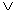# Translations of Encyclopedia about Mathematics

A dilemma usually refers to a difficult situation where a solution exists but where each chosen solution is usually equally unfeasible.

A dilemma usually takes the following form:

Either A or B

If A, then R

## If B, then S

Either R or S

A